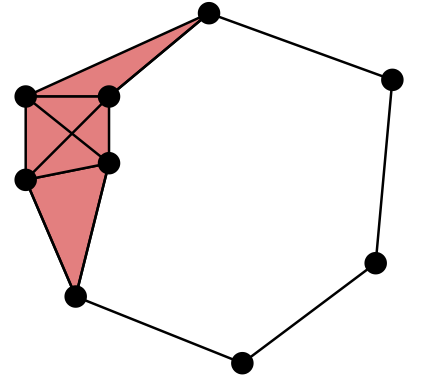# Vietoris–Rips Complexes¶Dionysus can compute Vietoris–Rips complexes. Given a point set $$P$$, a Vietoris–Rips complex consists of all those simplices whose vertices are at pairwise distance no more than $$r$$, $$VR_r(P) = \{ \sigma \subseteq P \mid \forall~u,v \in \sigma, \| u - v \| \leq r \}$$.

fill_rips() computes Vietoris–Rips filtrations (up to a specified skeleton dimension and distance $$r$$). It accepts points as NumPy arrays, following the standard convention that rows of a 2-dimensional array are interpreted as points in Euclidean space:

>>> import numpy as np
>>> points = np.random.random((100,2))
>>> f = d.fill_rips(points, 2, .3)
>>> print(f)
Filtration with 5974 simplices
>>> for s in f:
...     print(s)
<0> 0
...
<9,61,92> 0.299856
<9,72,92> 0.299856
<9,82,92> 0.299856


fill_rips() also accepts condensed distance matrices (linearized lower triangular part of a symmetric matrix):

>>> from scipy.spatial.distance import pdist
>>> dists = pdist(points)
>>> f = d.fill_rips(dists, 2, .3)
>>> print(f)
Filtration with 5974 simplices


SciPy provides a helper function squareform to convert between redundant square matrices ($$n \times n$$) and condensed matrices (vectors with $${n \choose 2}$$ elements).

>>> from scipy.spatial.distance import squareform
>>> sq_dist = squareform(dists)
>>> print(sq_dist.shape)
(100, 100)
>>> print(squareform(sq_dist).shape)
(4950,)